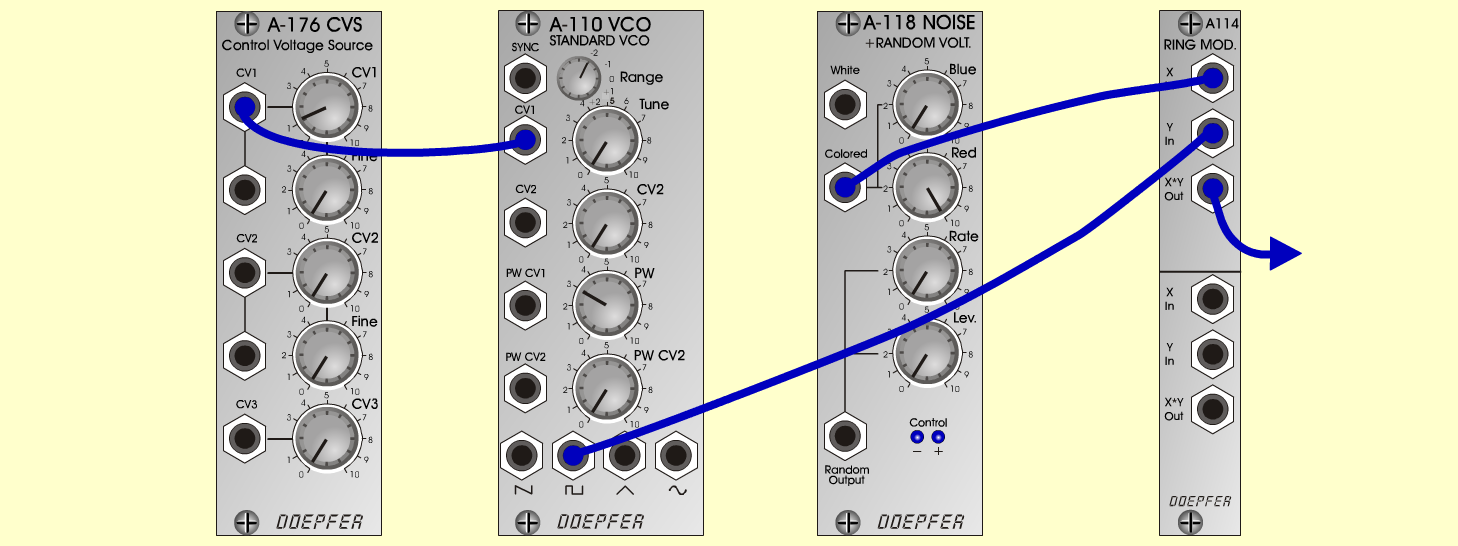Old MachineFXD o e p f e r A - 100 Connections: Settings: A-118 (Colored) <=> A-114 (X In) A-176 (CV 1) <=> A-110 (CV 1) A-110 (Pulse) <=> A-114 (Y In) A-114 (Out) <=> Amplifier A-110 (Range = -2, Tune = 0, PW = 3) A-118 (Blue = 0, Red = 10) A-176 (CV 1 = 1.2, Fine = 0) Josef MuellerSound samples Oldmachine (8 Hz) Oldmachine (13 Hz) Oldmachine (20 Hz) Oldmachine (30 Hz)